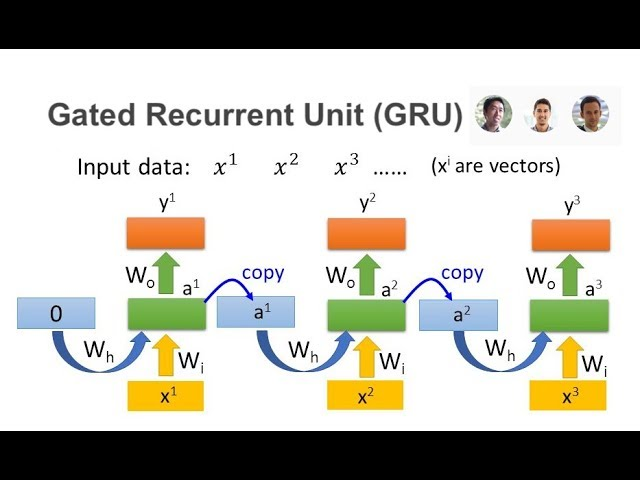# What is Gated recurrent units

##### Gated Recurrent Units: An Overview
Introduction: Gated Recurrent Units (GRU) are a type of recurrent neural network (RNN) that are used for processing sequential data. GRU was introduced in 2014 by Kyunghyun Cho et al. The GRU architecture is similar to Long Short-Term Memory (LSTM) networks, with fewer parameters, making them easier to train. In this article, we will discuss the GRU architecture and how it functions.

##### What are Recurrent Neural Networks (RNN)?
Recurrent Neural Networks (RNN) are a type of neural network that is used to process sequential data, where the output of the previous step is used as input for the current step. Unlike feedforward networks, RNNs can handle variable-length sequences of inputs. RNNs are commonly used in natural language processing (NLP) tasks such as text classification, language translation, and speech recognition.

##### GRU Architecture
The GRU architecture is similar to the LSTM architecture, but with fewer parameters. A GRU cell has two gates: reset gate and update gate. The reset gate determines how much of the previous state should be ignored, while the update gate determines how much of the new state should be retained. The general equations for a GRU cell are given below. -
Update Gate: zt = σ(Wz . [ht-1, xt] + bz) -
Reset Gate: rt = σ(Wr . [ht-1, xt] + br) -
Candidate Activation: h̃t = tanh(Wh . [rt * ht-1, xt] + bh) -
Hidden State: ht = (1 - zt) * ht-1 + zt * h̃t
Here, xt is the input at time step t,
ht-1 is the previous hidden state,
and ht is the current hidden state.
Wz, Wr, Wh, bz, br, and bh are weight matrices and bias vectors that are learned during training.
σ is the sigmoid activation function,
and * denotes element-wise multiplication.

##### How GRU works
The GRU architecture uses the reset gate to determine how much of the previous state should be ignored. If the reset gate is close to 0, then the previous state is ignored, and if it is close to 1, then the previous state is retained. The update gate determines how much of the new state should be retained. If the update gate is close to 0, then the new state is ignored, and if it is close to 1, then the new state is retained. The candidate activation is calculated using the previous hidden state and the current input. The reset gate is used to determine how much of the previous hidden state should be used for the candidate activation. The candidate activation is a new proposed hidden state, which is a combination of the current input and the previous hidden state that has been adjusted by the reset gate. The hidden state is calculated as a combination of the previous hidden state and the candidate activation, determined by the update gate. If the update gate is close to 0, then the previous hidden state is retained, and if it is close to 1, then the candidate activation is retained.

1. Fewer Parameters: The number of parameters in a GRU network is fewer than LSTM network, making them easier to train.
2. Faster Training: GRU networks are faster to train compared to LSTM networks, due to fewer parameters.
3. Better Performance: GRU networks can achieve similar performance as LSTM networks, while being faster to train.
4. Handles Long-Term Dependencies: GRU networks can handle long-term dependencies, making them suitable for processing sequential data.

##### Applications of GRU
1. Natural Language Processing: GRU networks are commonly used in natural language processing tasks such as text classification, language translation, and speech recognition.
2. Sequential Data Processing: GRU networks can be used for processing any kind of sequential data such as music, images, or stock prices.
3. Time Series Analysis: GRU networks can be used for analyzing time series data, such as predicting stock prices or detecting anomalies in sensor data.

##### Conclusion
Gated Recurrent Units (GRU) are a type of recurrent neural network that are used for processing sequential data. The GRU architecture is similar to the LSTM architecture, but with fewer parameters, making them easier to train. GRU networks can achieve similar performance as LSTM networks, while being faster to train. GRU networks can handle long-term dependencies, making them suitable for processing sequential data. GRU networks are commonly used in natural language processing, sequential data processing, and time series analysis.